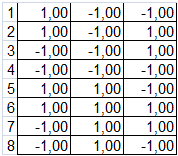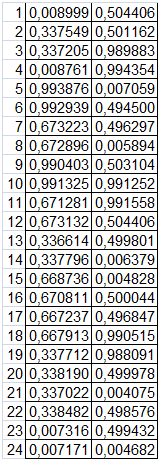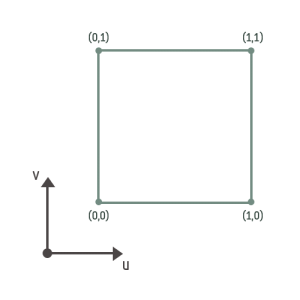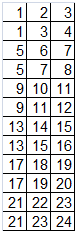This is the continuation of the “Loading a 3D model for your Tizen Native application - part 1” article. If you haven’t yet, please, get familiar with the first part before reading this one. The first article describes how to export a 3D model from Blender using the OBJ file format and how to understand this format. This one – how we implemented an example of the OBJ file format parser, which produces arrays necessary to draw the model in a 3D graphic application.

## How to get the necessary information from an OBJ file?

First, we need to define the path to our OBJ file:

```// objloader.h

It's used as the first argument of the getDataModelSizes() function. The second argument is the structure below:

```typedef struct _model_s
{
int vertices;
int positions;
int texels;
int faces;
};```

Having these numbers, we can allocate the memory for arrays that will store the data necessary to draw a model:

```   vertices = calloc(diceModel.vertices * 2, sizeof(float));
index_buffer = calloc(diceModel.vertices, sizeof(unsigned char));
texture_coord = calloc(diceModel.texels * 2, sizeof(float));```

To get the proper sizes we use the function below:

```void
getDataModelSizes(char* path, _model_s *model)
{
FILE *file = fopen(path, "r");
if (file == NULL) {
LOGE("ERROR OPENING OBJ FILE");
exit(1);
} else {
char line; /* or other suitable maximum line size */
char *result = fgets(line, sizeof line, file);

while (result != NULL ) /* read a line */
{
if (eina_str_has_prefix(line, "v "))
model->positions++;
else if (eina_str_has_prefix(line, "vt "))
model->texels++;
else if (eina_str_has_prefix(line, "f "))
model->faces++;
result = fgets(line, sizeof line, file);
}
fclose(file);
}
model->vertices = model->faces * 3;
}```

The only thing this does is count the number of lines starting with “v”, “vt” and “f” respectively. The created arrays we use as arguments of the getDataModel() function that gets data for our 3D model:

`getDataModel(fp, vertices, index_buffer, texture_coord, diceModel);`

```void
getDataModel(char* path, float *v, unsigned short *iB, float *tc, _model_s model)
{
float positions[model.positions];
float texels[model.texels];
int faces[model.faces];

int posIndex = 0;
int texIndex = 0;
int faceIndex = 0;
int faceIndex2 = 0;

char **arr;
char **arr2;
int i, j;

FILE *file = fopen(path, "r");

/* Read data from the file */
if (file == NULL) {
LOGE("ERROR OPENING OBJ FILE");
exit(1);
} else {
char line; /* or other suitable maximum line size */
char *result = fgets(line, sizeof line, file);

while (result != NULL ) /* read a line */
{
if (eina_str_has_prefix(line, "v ")) {
/* Vertices positions */
arr = eina_str_split(line, " ", 0);
positions[posIndex] = atof(arr);
positions[posIndex] = atof(arr);
positions[posIndex] = atof(arr);

posIndex++;
free(arr);
free(arr);
} else if (eina_str_has_prefix(line, "vt ")) {
/* Texture coordinates */
arr = eina_str_split(line, " ", 0);
texels[texIndex] = atof(arr);
texels[texIndex] = atof(arr);

texIndex++;
free(arr);
free(arr);
} else if (eina_str_has_prefix(line, "f ")) {
/* Faces */
arr = eina_str_split(line, " ", 0);
faceIndex2 = 0;
for (i = 1; arr[i]; i++) {
arr2 = eina_str_split(arr[i], "/", 0);
for (j = 0; arr2[j]; j++) {
faces[faceIndex][faceIndex2] = atoi(arr2[j]);
faceIndex2++;
}
}
faceIndex++;

free(arr2);
free(arr2);
free(arr);
free(arr);
}
result = fgets(line, sizeof line, file);
}
fclose(file);
}```

We have read data from the .obj file. It can be saved in the form of three matrices:

positions[][] - a matrix storing subsequent coordinates of cube vertices:texels[][] - a matrix storing subsequent texture pixels:faces[][] - a matrix storing the corresponding indices from both matrices (positions and texels):What does it mean? That we have twelve faces of our dice. The grey columns store vertex indices, white – texture pixels indexes. We use this data to create the index_buffer array.

The gray columns store the indices of elements from a cube vertices array, which constitute a given face; the white ones are the indices of elements from a texture coordinates array. Data from the faces[][] matrix is used to create an indices array (index_buffer).

Let us note two things:

• We need only one table of indices pointing to specific coordinates of vertices or textures. This means that an element of the indices array with the value of “22” indicates the twenty-second element of the texture coordinates array and the twenty-second element of the vertices array (!). So, the number of vertices array elements must be the same as the number of texture coordinates array elements. The twenty-second element of the vertices array is equal to the first element of the vertices array.
• The values in the above matrix starts from the ones, but array elements must be numbered from zero.

As a result we make the following transformation of our data:

```      /* Format data in a proper way */
float vertices[texIndex];

for (i = 0; i < faceIndex; i++) {
for (j = 0; j < 3; j++) {
vertices[faces[i] - 1][j] = positions[faces[i] - 1][j];
vertices[faces[i] - 1][j] = positions[faces[i] - 1][j];
vertices[faces[i] - 1][j] = positions[faces[i] - 1][j];
}
}

int k = 0;

for (i = 0; i < 24; i++)
for (j = 0; j < 3; j++) {
v[k++] = vertices[i][j];
}```

We create a new vertex matrix with the size equal to the number of texture coordinates array elements multiplied by 3. We assign a corresponding element of the vertices array for each element. Next, we copy the elements of the newly created array line by line to a vertices array (v). Of course, it could be done in one loop, but it would be less legible.

Regarding the texture coordinates matrix: we rewrite it line by line to the texture coordinates array.

```      k = 0;

for (i = 0; i < 24; i++)
for (j = 0; j < 2; j++) {
tc[k++] = texels[i][j];
}```

In case of the faces matrix we rewrite the elements from column with odd index (white column) line by line:

```      for (i = 0; i < 12; i++) {
for (j = 0; j < 3; j++) {
iB[3 * i + j] = (unsigned short) (faces[i][2 * j + 1] - 1);
}
}
```

As result:We already made sure that they correspond to the vertices array elements.

The vertices, texture_coord and index_buffer arrays are used to draw our 3D model:

```static void
draw_model(Evas_Object *obj)
{
static float zPos = -5.0f;
static float zPosInc = Z_POS_INC;
static int angle = 0;

ELEMENTARY_GLVIEW_USE(obj);

glEnableClientState(GL_VERTEX_ARRAY);
glVertexPointer(3, GL_FLOAT, 0, vertices);

glEnableClientState(GL_TEXTURE_COORD_ARRAY);
glTexCoordPointer(2, GL_FLOAT, 0, texture_coord);

glEnable(GL_TEXTURE_2D);
glMatrixMode(GL_MODELVIEW);

zPos += zPosInc;
if (zPos < -8.0f)
{
zPosInc = Z_POS_INC;
}
if (zPos > -5.0f)
zPosInc = -Z_POS_INC;

glTranslatef(0, 1.2f, zPos);

angle = (angle + 5) % (360 * 3);
glRotatef((float)angle, 0, 1.0f, 0);
glRotatef((float)angle, 1.0f, 0, 0);

glDrawElements(GL_TRIANGLES, diceModel.vertices, GL_UNSIGNED_SHORT, &index_buffer);

glDisable(GL_TEXTURE_2D);
glDisableClientState(GL_VERTEX_ARRAY);
glDisableClientState(GL_TEXTURE_COORD_ARRAY);
}```

Note: Drawing a 3D model using OpenGL ES 1.1 isn't in the scope of this article.

첨부 파일:dice.pngdice.zipobjloader.zip
List
SDK Version Since:
2.3.1